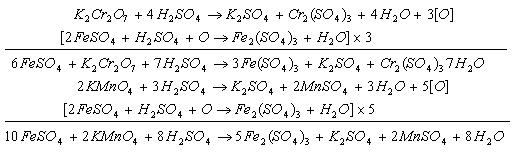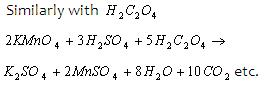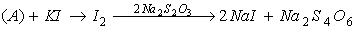1800-1023-196

+91-120-4616500

# Volumetric Analysis

## Volumetric Analysis

It is a method which involves quantitative determination of the amount of any substance present in a solution through volume measurements. For the analysis a standard solution is required. (A solution which contains a known weight of the solute present in known volume of the solution is known as standard solution.)

To determine the strength of unknown solution with the help of known (standard) solution is known as titration. Different types of titrations are possible which are summerised as follows,

(i) Redox titrations: To determine the strength of oxidising agents or reducing agents by titration with the help of standard solution of reducing agents or oxidising agents.

Examples:(ii) Acid-base titrations: To determine the strength of acid or base with the help of standard solution of base or acid.(iii) Iodiometric titrations: This is a simple titration involving free iodine. This involves the titration of iodine solution with known sodium thiosulphate solution whose normality is N. Let the volume of sodium thiosulphate is Vml.
I2 + 2Na2S2O3 → 2 NaI + Na2S4O6

n = 2, n = 1

Equivalents of I2 Equivalent of Na2S2O3

Equivalents of I2 = N × V × 10-3

Moles of I2 = N × V×10-3/2

Mass of free I2 in the solution = [(N×V×10-3/2)×254]g.

(iv) Iodometric titrations: This is an indirect method of estimation of iodine. An oxidising agent is made to react with excess of solid Kl. The oxidising agent oxidises I- to I2. This iodine is then made to react with Na2S2O3 solution.

Oxidising AgentLet the normality of Na2S2O3 solution is N and the volume of thiosulphate consumed to Vml.

Equivalent of A= Equivalent of I2 Equivalents of Na2S2O3

Equivalents of I2 liberated from Kl = N×V×10-3

Moles of I2 liberated from Kl = (N×V×10-3/2)

Mass of I2 liberated from Kl = [(N×V×10-3/2)×254]g.

## NEET & AIIMS Exam Sample Papers

 AIIMS SAMPLE PAPERS View More NEET SAMPLE PAPERS View More